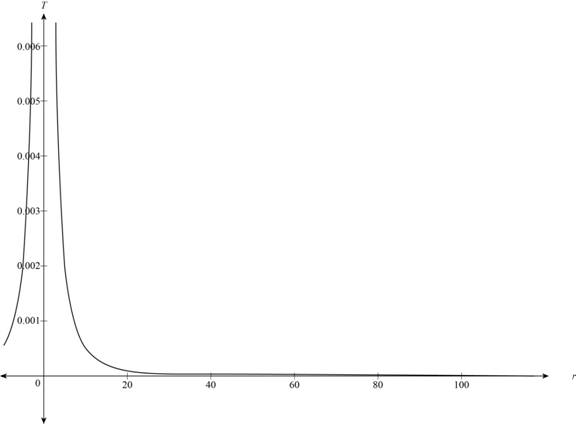# The graph for the given function.### Precalculus: Mathematics for Calcu...

6th Edition
Stewart + 5 others
Publisher: Cengage Learning
ISBN: 9780840068071### Precalculus: Mathematics for Calcu...

6th Edition
Stewart + 5 others
Publisher: Cengage Learning
ISBN: 9780840068071

#### Solutions

Chapter 2.2, Problem 79E
To determine

## To sketch: The graph for the given function.

Expert Solution

### Explanation of Solution

Relation between the thickness (T) and radius (r) of the inflated balloon is,

T(r)=(0.5r2)

The value of r is lie between 10 and 100.

Substitute 10 for r in the given function,

T(r)=(0.5r2)=(0.510×10)=(0.005×100)(1m=100cm)T(10)=0.5cm

Substitute 100 for r in the given function,

T(r)=(0.5r2)=(0.5100×100)=(0.00005×100)(1m=100cm)T(100)=0.005cm

The range of thickness and radius lies from (0.005cm) to 100 respectively as shown in below graph.

The graph is,Figure (1)

Thus, the above graph shows the relation between the thickness (T) and radius (r) of the weather inflated balloon.

### Have a homework question?

Subscribe to bartleby learn! Ask subject matter experts 30 homework questions each month. Plus, you’ll have access to millions of step-by-step textbook answers!